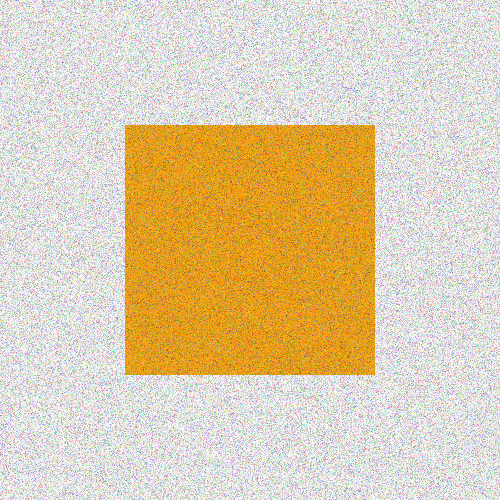# Placing images

Luxor lets you place existing images on the drawing. You can place PNG, SVG, and EPS images. (JPEGs aren't supported.)

For PNG and SVG, first load the image and create a reference to it. For example:

• for PNG images, use img = readpng(filename)
• for SVG images, use img = readsvg(filename) or img = readsvg(string)

You can access this image's dimensions with img.width and img.height.

Use placeimage(img) to place the image by its top left corner at point pt. Use the centered=true keyword to place the image's center point there.

img = readpng("../assets/figures/julia-logo-mask.png")
w = img.width
h = img.height
rulers()
scale(0.3, 0.3)
rotate(π/4)
placeimage(img, Point(-w/2, -h/2), .5)
sethue("red")
circle(-w/2, -h/2, 15, :fill)PNG images can be placed with varying opacity or transparency. For example, the image is placed with 0.5 opacity with:

placeimage(img, Point(100, 100), 0.5)

SVG images do their own thing in terms of opacity.

readsvg also lets you supply raw (or pure) SVG code in a string.

You can use placeimage() to place an array of RGB or RGBA pixels on a drawing.

N = 500
i = reshape([RGBA(rand(4)...) for p in 1:N^2], N, N)
# i is Matrix{RGBA{Float64}}
# (alias for Array{RGBA{Float64}, 2})
@draw begin
origin()
sethue("orange")
box(O, N/2, N/2, :fill)
placeimage(i, O, centered=true, alpha=0.5)
end 500 500Or you can load an image as an array and place it on a drawing.

using Luxor, Colors, FileIO
@draw begin
img[1:50, :] .= colorant"cyan"
img[200:end, :] .= colorant"magenta"
placeimage(img, O, centered=true, alpha=0.5)
end 250 250## SVG images

To output a drawing as an SVG image, using the Drawing(... :svg) or specify an SVG filename. To obtain the SVG source of a completed SVG drawing, use svgstring.

For example, if you draw the Julia logo like this:

Drawing(500, 500, :svg)
origin()
julialogo()
finish()
s = svgstring()

You'll get the SVG source code stored, as a string, in s. You can examine or process it further. For example, the five colors used for the logo were:

eachmatch(r"rgb\$$.*?\$$", s) |> collect
5-element Vector{RegexMatch}:
RegexMatch("rgb(0%,0%,0%)")
RegexMatch("rgb(79.6%,23.5%,20%)")
RegexMatch("rgb(25.1%,38.8%,84.7%)")
RegexMatch("rgb(58.4%,34.5%,69.8%)")
RegexMatch("rgb(22%,59.6%,14.9%)")

To display the image in a Jupyter or Pluto notebook, use the HTML function, or you can use the readsvg and placeimage functions in combination.

## EPS images

EPS (Encapsulated PostScript) files created by Luxor (or any Cairo-based package) can be re-imported and placed on the current drawing with the placeeps function. This function converts the EPS commands to the equivalent Luxor commands and evaluates them immediately in the context of the current drawing.

Warning

This function is designed to extract just the coordinates of paths from an EPS file. An EPS file can contain much more information about an image than coordinates: there migth be image and pixel data, font data, linear color gradients, and so on. These are not translated into equivalent Luxor functions. This function interprets the EPS commands in a Cairo-generated EPS "Prolog"; EPS files created by other applications will likely not contain this Cairo-generated Prolog, and so won't be interpreted at all (or will go wrong in interesting ways).

In this example, an SVG file linnux.svg is placed and exported to an EPS file linux.eps, then this EPS file is imported and placed on a new SVG drawing using Luxor functions instead of EPS commands, respecting the current scale and orientation. Finally, when the SVG document is finished, the graphics will be in SVG format again.

using Luxor

svgfile = dirname(@__FILE__) * "../assets/figures/linux.svg"
epsfile = dirname(@__FILE__) * "../assets/figures/linux.eps"

@eps begin
placeimage(img, centered = true)
end 500 500 epsfile

@drawsvg begin
translate(midpoint(boxtopleft(), O))
scale(0.5)
rotate(π/12)
placeeps(epsfile)
rulers()
endIf you want to obtain the paths and coordinates for use elsewhere, you can use the log=true function, which sends the commands to the REPL as well:

placeeps("/tmp/linux.eps", log=true)

# start EPS import
gsave()
setgray(1.0)
move(Point(70.801, -6.0))
line(Point(429.199, -6.0))
curve(Point(471.617, -6.0), Point(506.0, 28.383), Point(506.0, 70.801))
line(Point(506.0, 429.199))
curve(Point(506.0, 471.617), Point(471.617, 506.0), Point(429.199, 506.0))
line(Point(70.801, 506.0))
curve(Point(28.383, 506.0), Point(-6.0, 471.617), Point(-6.0, 429.199))
line(Point(-6.0, 70.801))
curve(Point(-6.0, 28.383), Point(28.383, -6.0), Point(70.801, -6.0))
closepath()
move(Point(70.801, -6.0))
fillpath()
...

Once you have a sequence of Luxor commands, you can edit them into new creations:## Placing an image matrix

You can use placeimage to put pixel images on a drawing.

This example uses noise to define the RGB values in a matrix of ARGB32 color values:

D = 600
mat = [Luxor.ARGB32(
noise(0.01r, 0.01c),
noise(0.1r, 0.02c),
noise(0.1r, 0.01c)) for r in 1:D, c in 1:D]

@drawsvg begin
placeimage(mat, centered=true)
fontsize(80)
sethue("white")
setopacity(0.5)
text("woah", halign=:center)
end D D÷2